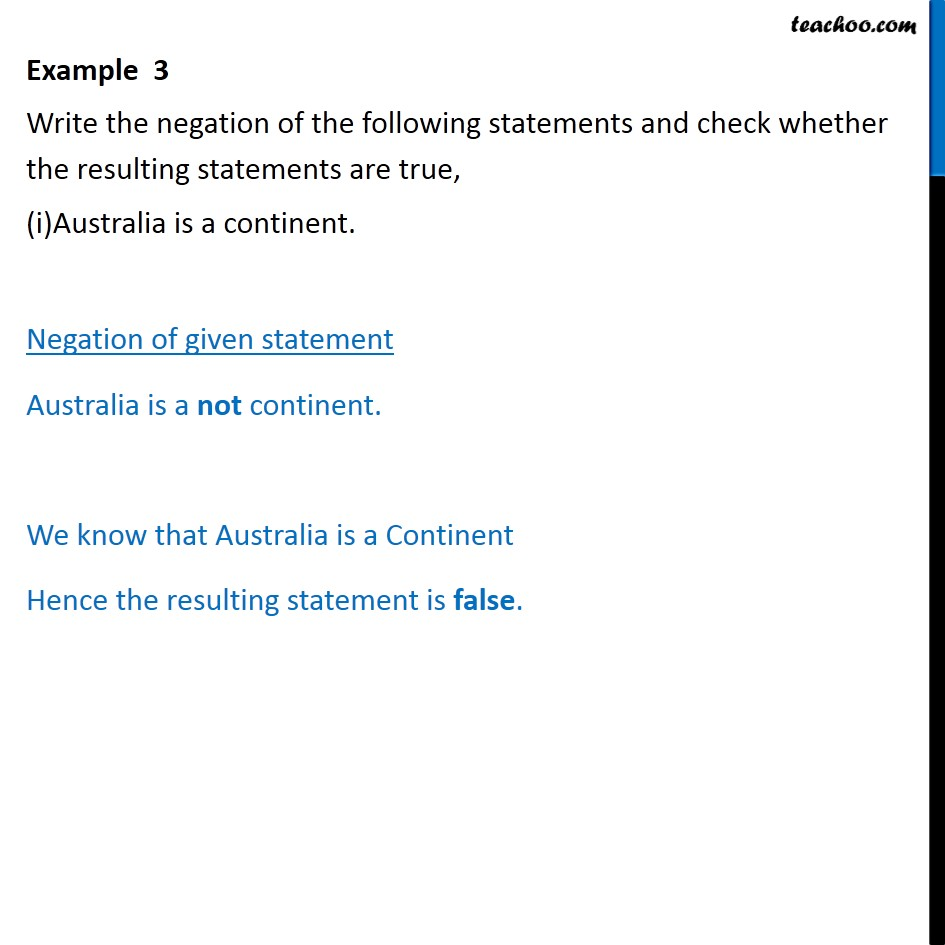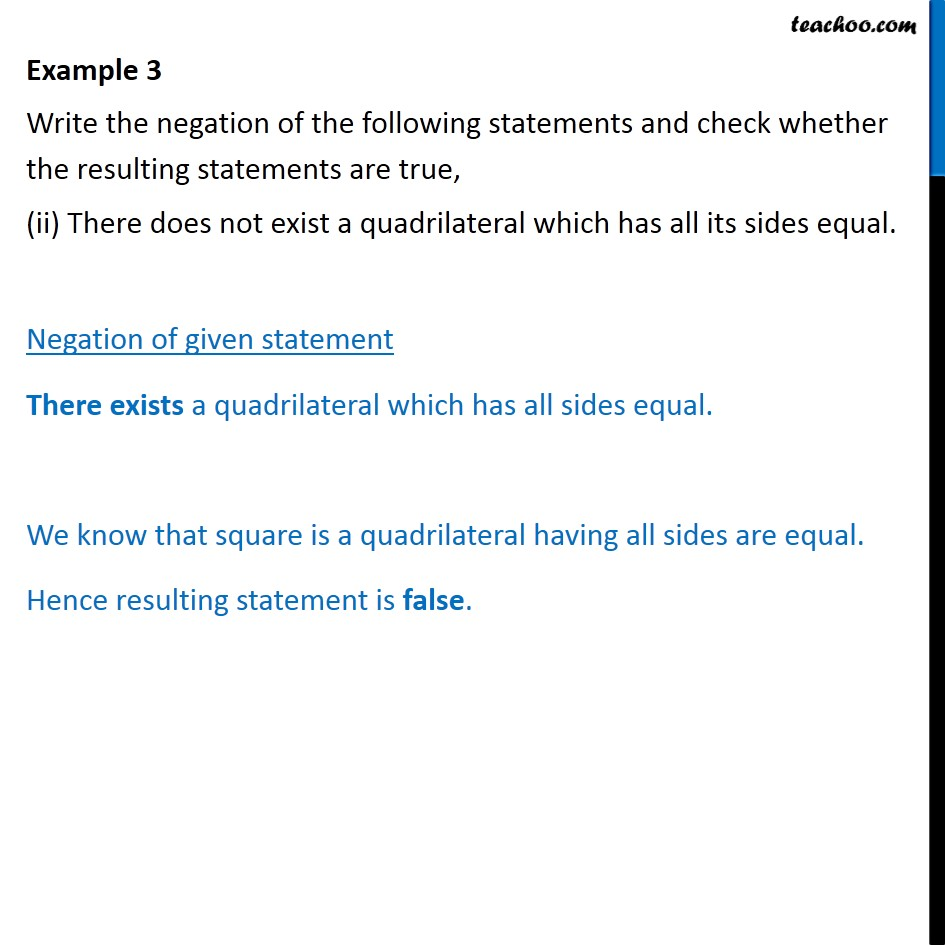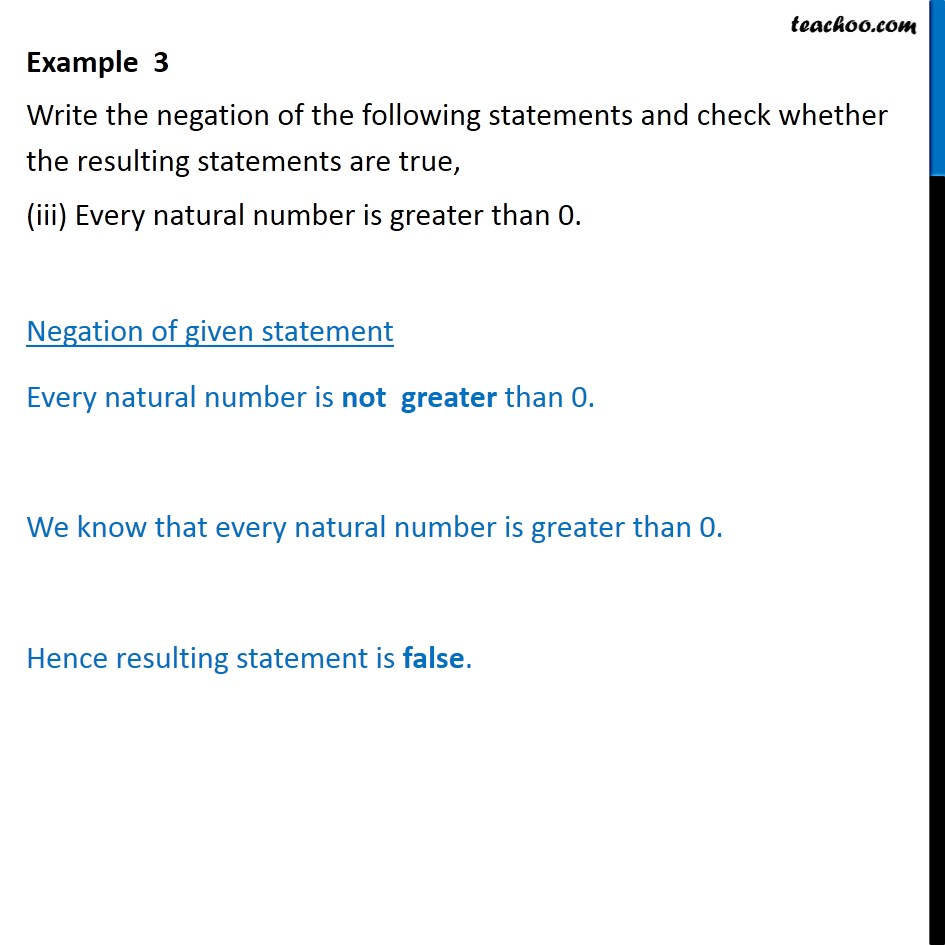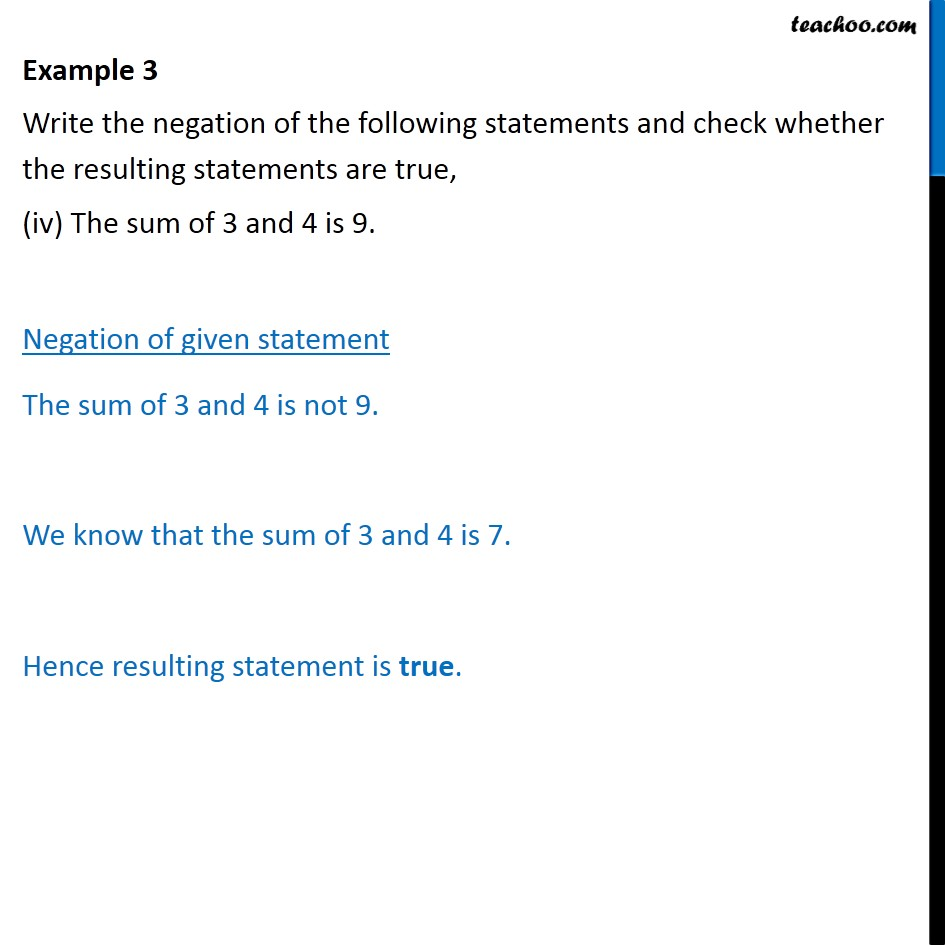Subscribe to our Youtube Channel - https://you.tube/teachoo

1. Chapter 14 Class 11 Mathematical Reasoning
2. Concept wise
3. Negation - Checking if true or not

Transcript

Example 3 Write the negation of the following statements and check whether the resulting statements are true, (i)Australia is a continent. Negation of given statement Australia is a not continent. We know that Australia is a Continent Hence the resulting statement is false. Example 3 Write the negation of the following statements and check whether the resulting statements are true, (ii) There does not exist a quadrilateral which has all its sides equal. Negation of given statement There exists a quadrilateral which has all sides equal. We know that square is a quadrilateral having all sides are equal. Hence resulting statement is false. Example 3 Write the negation of the following statements and check whether the resulting statements are true, (iii) Every natural number is greater than 0. Negation of given statement Every natural number is not greater than 0. We know that every natural number is greater than 0. Hence resulting statement is false. Example 3 Write the negation of the following statements and check whether the resulting statements are true, (iv) The sum of 3 and 4 is 9. Negation of given statement The sum of 3 and 4 is not 9. We know that the sum of 3 and 4 is 7. Hence resulting statement is true.

Negation - Checking if true or not